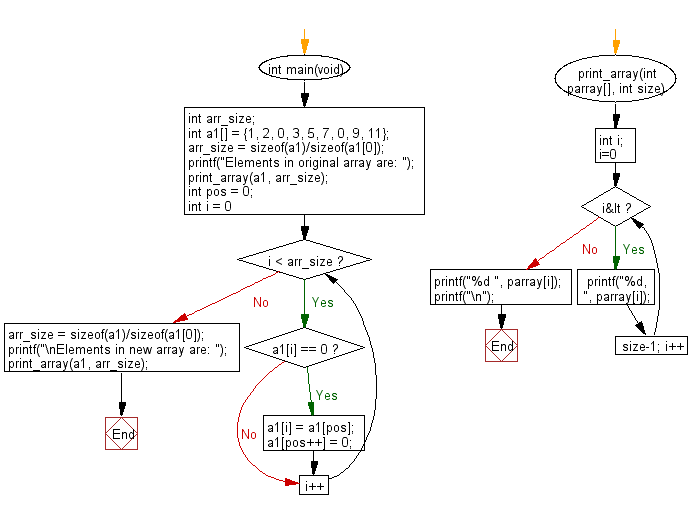﻿ C exercises: Create a new array from a given array of integers shifting all zeros to left direction - w3resource# C Exercises: Create a new array from a given array of integers shifting all zeros to left direction

## C-programming basic algorithm: Exercise-71 with Solution

Write a C program to create a new array from a given array of integers shifting all zeros to left direction.

C Code:

``````#include <stdio.h>
#include <stdlib.h>
int main(void){
int arr_size;
int a1[] = {1, 2, 0, 3, 5, 7, 0, 9, 11};
arr_size = sizeof(a1)/sizeof(a1);
printf("Elements in original array are: ");
print_array(a1, arr_size);
int pos = 0;
for (int i = 0; i < arr_size; i++)
{
if (a1[i] == 0)
{
a1[i] = a1[pos];
a1[pos++] = 0;
}
}

arr_size = sizeof(a1)/sizeof(a1);
printf("\nElements in new array are: ");
print_array(a1, arr_size);
}
print_array(int parray[], int size)
{
int i;
for( i=0; i<size-1; i++)
{
printf("%d, ", parray[i]);
}
printf("%d ", parray[i]);
printf("\n");
}
``````

Sample Output:

```Elements in original array are: 1, 2, 0, 3, 5, 7, 0, 9, 11

Elements in new array are: 0, 0, 1, 3, 5, 7, 2, 9, 11
```

Pictorial Presentation:Flowchart:C Programming Code Editor: